# Cardboard box

Peter had square cardboard. The length of the pages was an integer in decimetres. He cut four squares with a side of 3 dm from the corners and made a box out of it, which fit exactly 108 cubes with an edge 1 dm long.
Julia cut four squares with a side of 2 dm from the corners of the same cardboard and created a box. He wants to put cubes with an edge of 2 dm in it. How many cubes will fit in Julia's box?

n =  16

### Step-by-step explanation: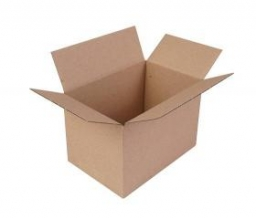Did you find an error or inaccuracy? Feel free to write us. Thank you!Tips to related online calculators
Do you want to convert area units?
Do you know the volume and unit volume, and want to convert volume units?

#### You need to know the following knowledge to solve this word math problem:

We encourage you to watch this tutorial video on this math problem:

## Related math problems and questions:

• Cardboard boxWe want to make a cardboard box shaped quadrangular prism with rhombic base. Rhombus has a side of 5 cm and 8 cm one diagonal long. The height of the box to be 12 cm. The box will be open at the top. How many square centimeters cardboard we need, if we ca
• Three cubes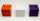The body was created by gluing three identical cubes. Its volume is 192 cm3. What is its surface in dm2?
• Cube 9What was the cube's original edge length after cutting 39 small cubes with an edge of 2 dm left 200 dm3?
• The coilHow many ropes (the diameter 8 mm) fit on the coil (threads are wrapped close together) The coil has dimension: the inner diameter 400mm, the outside diameter 800mm and the length of the coil is 470mm
• The body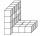The body on the figure consists of cubes with an edge length 10 cm. What surface has this body?
• Floating barrelBarrel (cylinder shape) floats on water, top of the barrel is 8 dm above water, and the width of surfaced barrel part is 23 dm. Barrel length is 24 dm. Calculate the volume of the barrel.
• Squares ratioThe first square has a side length of a = 6 cm. The second square has a circumference of 6 dm. Calculate the proportions of the perimeters and the proportions of the contents of these squares? (Write the ratio in the basic form). (Perimeter = 4 * a, conte
• Cube corners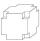A small cube with an edge length of 2 cm was cut from each corner of a large cube with an edge length of 10 cm. How many cm3 was the body left from the big cube after cutting the small cubes?
• BoxCardboard box-shaped quadrangular prism with a rhombic base. Rhombus has a side 5 cm, and one diagonal 8 cm long, and the box's height is 12 cm. The box will open at the top. How many cm2 of cardboard do we need to cover overlap and joints that are 5% of
• CubesHow many cubes with an edge length of 5 cm may fit in the cube with an inner edge of 0.4 m maximally?
• Paper boxThe hard rectangular paper has dimensions of 60 cm and 28 cm. The corners are cut off equal squares, and the residue was bent to form an open box. How long must beside the squares be the largest volume of the box?
• PrismCalculate the height of the prism having a surface area 448.88 dm² wherein the base is square with a side of 6.2 dm. What will be its volume in hectoliters?
• TentCalculate how many liters of air will fit in the tent that has a shield in the shape of an isosceles right triangle with legs r = 3 m long the height = 1.5 m and a side length d = 5 m.
• Paper box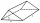Calculate how much we'll pay for a three-side shaped prism box with a triangular base, and if it measures 12cm and 1.6dm, the hypotenuse measures 200mm. The box is 34cm high. We pay 0,13 € per square meter of paper.
• CubesOne cube is an inscribed sphere and the other one described. Calculate the difference of volumes of cubes, if the difference of surfaces in 257 mm2.
• Square metal sheetWe cut out four squares of 300 mm side from a square sheet metal plate with a side of 0,7 m. Express the fraction and the percentage of waste from the square metal sheet.
• Cube-shaped boxThe cube-shaped box is filled to the brim with 2 liters of milk. Calculate the edge and surface of the box.